# Transfer Function in the Frequency Domain

   

Transfer function in the frequency domain of AC circuits are presented with examples and their solutions. Problems and their solutions are also included. Problems and their solutions are also included.
The ideas of using complex numbers in AC circuits and the computations of in RLC circuits are used to develop and compute transfer functions in the frequency domain.
Note that we usually express the impedances using $j \omega$. However, for more complicated expressions of the impedances, it is perhaps easier to use $s = j \omega$ to end up with simplified expressions.
More on transfer functions of cascaded circuits is included.

## A - Frequency Behaviour of Capacitors and Inductors Impedances

Capacitors and inductors behave differently to different frequencies.
At a given frequency $\omega$, the impedance $X_C$ of a capcitor with capacitance $C$ is given by $Z_C = \; \dfrac{1}{ j \; \omega \; C}$ and the impedance $X_L$ of an inductor with inductance $L$ is given by $Z_L = j \; \omega L$ Both $X_C$ and $X_L$ are impedances in complex forms and the modulus of each is given by $| Z_C | = \dfrac{1}{\omega \; C}$ $| Z_L | = \omega L$ Let $C = 100 \mu \; F$ and $L = 100 \; m H$ and graph $| Z_C |$ and $| Z_L |$
The graphs of $| Z_C |$ and $| Z_L |$ against the angular frequency $\omega$ are shown below. The graph of $|Z_C|$ is that of a hyperbola and that of $|Z_L|$ is that of a line.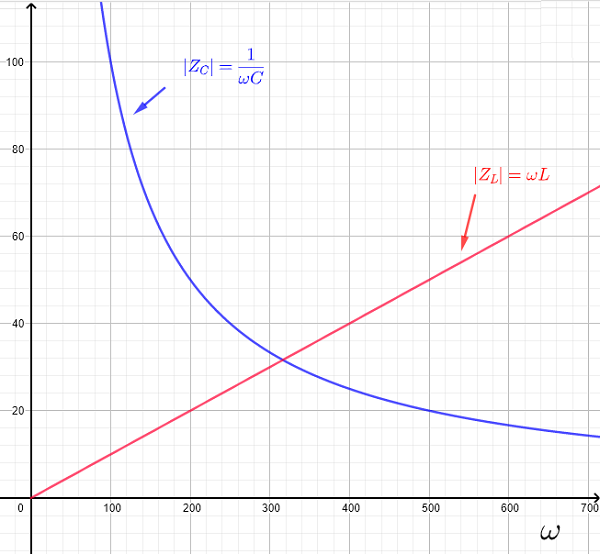Important properties to note:
1) As the frequency is small and close to zero, the impedance $|Z_C|$ of the capacitor is very large and the impedance $|Z_L|$ of the inductor is very small (close to zero).
2) As the frequency is large, the impedance $|Z_C|$ of the capacitor is very small (close to zero) and the impedance $|Z_L|$ of the inductor is large.
3) In general impedances including a combinations of resistances, capacitors and inductors are functions of the frequency and therefore voltages and currents are also functions of the frequency.
Also when an impedance is large, we can assume it bevhaves like an open circuit and when the impedance is small it behaves like a short circuit.
The above properties help us understand the properties of different ac circuits.
Note that if we write $s = j \omega$, the impedances of the of a capacitor with capaciatnce $C$ may be written as
$Z_C = \; \dfrac{1}{ s \; C}$ the impedances of the of an inductor with inductance $L$ may be written as $Z_L = s L$

## Review of Complex Numbers In Polar Form

In complex numbers, the imaginary unit is defined by $j = \sqrt {-1}$ or $j^2 = - 1$
The polar form of a complex number $Z = a + j b$ is given by
$Z = |Z| \; \angle \; \theta$
where $|Z|$ and $\theta$ are the modulus and argument , of $Z$, respectively and are defined by
$|Z| = \sqrt {a^2 + b^2}$ and $\theta = \arctan \left( \dfrac{b}{a} \right)$ within the range $-\pi \lt \theta \le \pi$
One of the main advantage in using complex numbers in polar form in electrocnic ac circuits is the ease of dividing and mutliplying these numbers.
Let two complex numbers $Z_1$ and $Z_2$ given in polar form as follows
$Z_1 = |Z_1| \; \angle \; \theta_1$ and $Z_2 = |Z_2| \; \angle \; \theta_2$
Product
The product of $Z_1$ and $Z_2$ are given by
$Z_1 \cdot Z_2 = |Z_1| \cdot |Z_2| \; \angle \; \theta_1 + \theta_2$
Division
The division of $Z_1$ and $Z_2$ are given by
$\dfrac{Z_1}{Z_2} = \dfrac{|Z_1|}{|Z_2|} \; \angle \; \theta_1 - \theta_2$
Power
$Z_1^n$ is given by
$Z_1^n = |Z_1|^n \angle \; n \theta_1$

## B-Voltage Transfer Function in the Frequency Domain

We consider the simple voltage divider below and use the voltages and impedances to express the output voltage as

Using Kirchhoff's and Ohm's law extended to AC circuits where $Z_1$ and $Z_2$ are complex impedances, we obtain
$V_{out} = \dfrac{Z_2}{Z_2+Z_1} V_{in}$

$\dfrac{V_{out}}{V_{in}} = \dfrac{Z_2}{Z_2+Z_1}$

where $V_{out}$ and $V_{in}$ are the complex form of the volatges $v_{out}$ and $v_{in}$.
In general $Z_1$ and $Z_2$ depend on the frequency $\omega$ of the voltage source $v_i$ and the ratio $H(\omega) = \dfrac{V_{out}}{V_{in}}$ is called the voltage transfer function in the frequency domain.
In the above example $H(\omega)$ is given by
$H(\omega) = \dfrac{Z_2}{Z_2+Z_1}$
The transfer function $H$ is a function of $\omega$ because in general the impedances are functions of the frequency of the source voltage (or current) as seen above.

Example 1
Find the transfer function in the frequency domain of the circuit below and graph it magnitude and argument (or phase).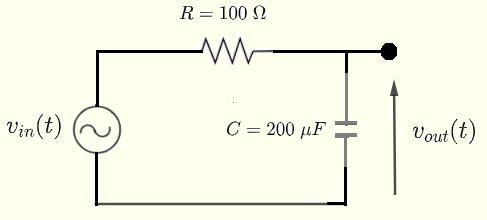Solution to Example 1
Using the formulas of impedances in ac circuits , in the RC circuit below, the output voltage (in complex form) $V_{out}$ is given by
$V_{out} = \dfrac{\; \dfrac{1}{ j \; \omega \; C} }{ \; \dfrac{1}{ j \; \omega \; C} + R } V_{in}$
Simplify the above and write the voltage transfer function $\dfrac{V_{out}}{V_{in}}$ in the frequency domain as follows

$H(\omega) = \dfrac{V_{out}}{V_{in}} = \dfrac {1}{1 + j \omega R \; C}$

$H(\omega)$ is a transfer function in the frequency domain since it gives a relationship between the output and the input and it depends on the frequency $\omega$. The transfer function in the frequency domain is a complex number and may be written in polar form which was reviewed above. $H(\omega) = | H(\omega) | \; \angle \phi(\omega)$ where $| H(\omega) |$ is the modulus (magnitude) of $H(\omega)$ and $\phi(\omega)$ is the argument (phase) of $H(\omega)$.
The denominator of $H(\omega) = \dfrac {1}{1 + j \omega R \; C}$ obtained above, may be written in polar form as
$1 = 1 \angle 0$
and the denominator may be written as
$1 + j \omega R \; C = \sqrt{1^2 + (\omega \; R \; C)^2} \; \angle \arctan(\omega \; R \; C)$
Hence using the division of complex numbers in polar form : $\dfrac{|z_1| \angle \phi_1 }{|z_2| \angle \phi_2 } = \dfrac{|z_1|}{|z_2|} \angle (\phi_1 - \phi_2)$ , we write $H(\omega)$ as follows
$H(\omega) = \dfrac{1}{\sqrt{{1 + (\omega \; R \; C)^2}}} \; \angle - \arctan(\omega \; R \; C)$
Use the numerical values of the capacitance and inductance given above, evaluate $R C = 100 \times 200 \times 10^{-6} = 0.02$
Hence
$H(\omega) = \dfrac{1}{\sqrt{{1 + 0.0004 \; \omega^2}}} \; \angle - \arctan(0.02 \; \omega)$

The graph of the magnitude of the transfer function given by the expression $20 \; \log_{10} \left(\dfrac{1}{\sqrt{{1 + 0.0004 \; \omega^2}}} \right)$ against the frequency omega is shown below.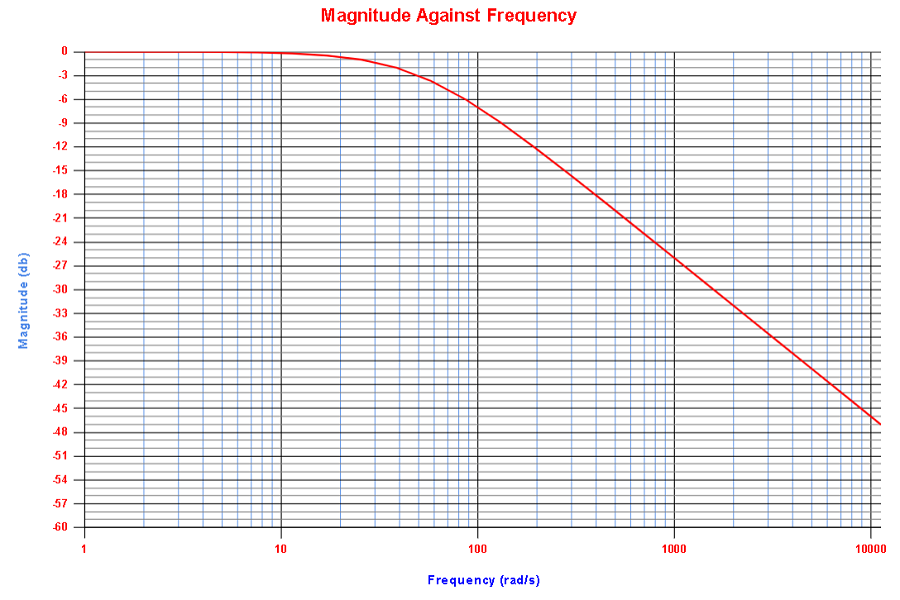The graph of the phase of the transfer function given by the expression $- \arctan(0.02 \; \omega)$ ( and converted in degrees) against the frequency omega is shown below.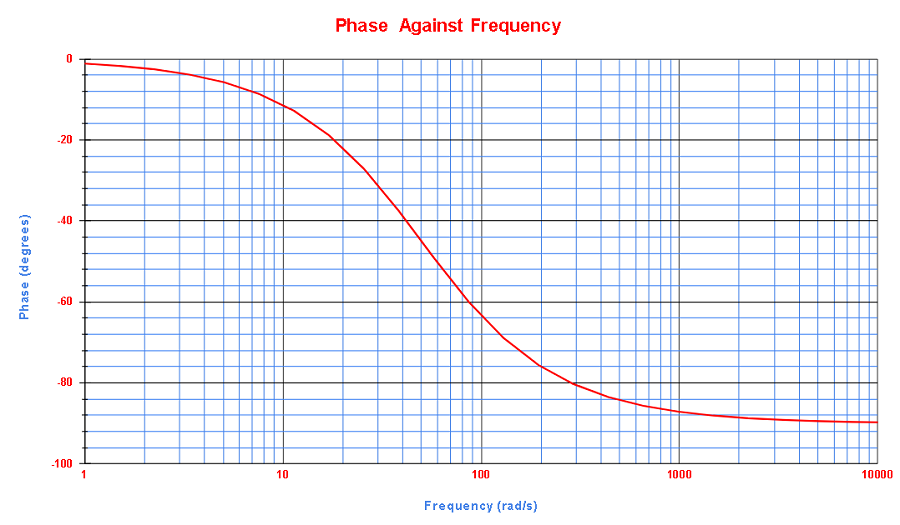Example 2
Find the transfer function in the frequency domain of the circuit below and graph it magnitude and argument (or phase).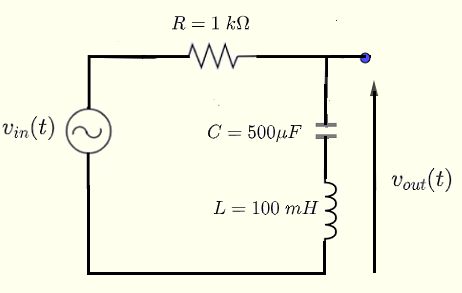Solution to Example 2
Using the formulas of impedances in ac circuits , in the RC circuit below, the output voltage (in complex form) $V_{out}$ is given by
$V_{out} = \dfrac{ \; \dfrac{1}{ j \; \omega \; C} + j \; L \; \omega}{ \; \dfrac{1}{ j \; \omega \; C} + j \; L \; \omega + R } V_{in}$

Multiply numerator and denominator by $j \; \omega \; C$ and simplify to obtain the voltage transfer function $\dfrac{V_{out}}{V_{in}}$ in the frequency as

$H(\omega) = \dfrac{1 - L \; C \; \omega^2 }{1 - L \; C \; \omega^2 + j \; R \; C \; \omega}$

The transfer function in the frequency domain may be written in polar form as $H(\omega) = | H(\omega) | \; \angle \phi(\omega)$ The magnitude $| H(\omega) |$ of $H(\omega)$ is given by

$| H(\omega) | = \dfrac{|1 - L \; C \; \omega^2 |}{\sqrt{ (1 - L \; C \; \omega^2 )^2 + (R \; C \; \omega)^2 }}$

The phase $\phi(\omega)$ of $H(\omega)$ is given by

$\phi(\omega) = - \arctan \left(\dfrac{R \; C \; \omega}{1 - L \; C \; \omega^2} \right)$
The graphs of $\; 20 \log_{10} | H(\omega) |$ and the phase $\phi(\omega)$ are shown below.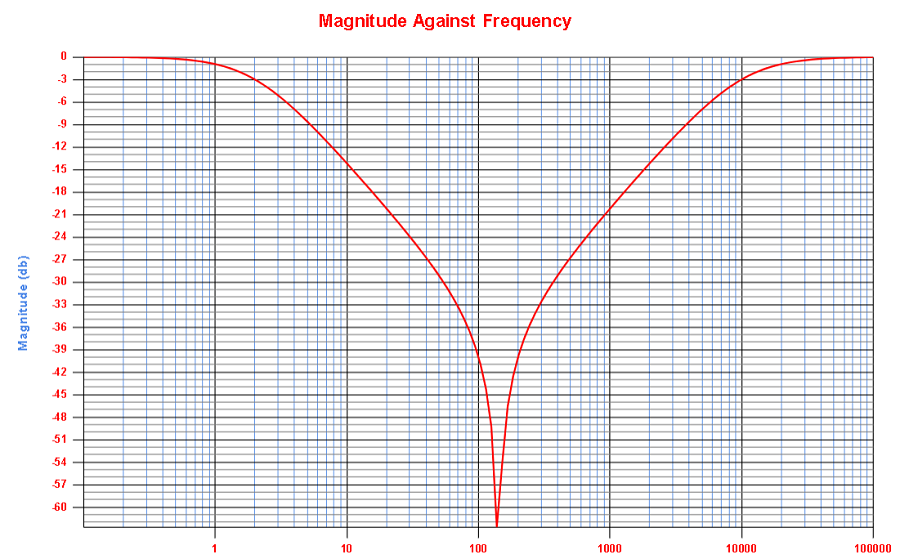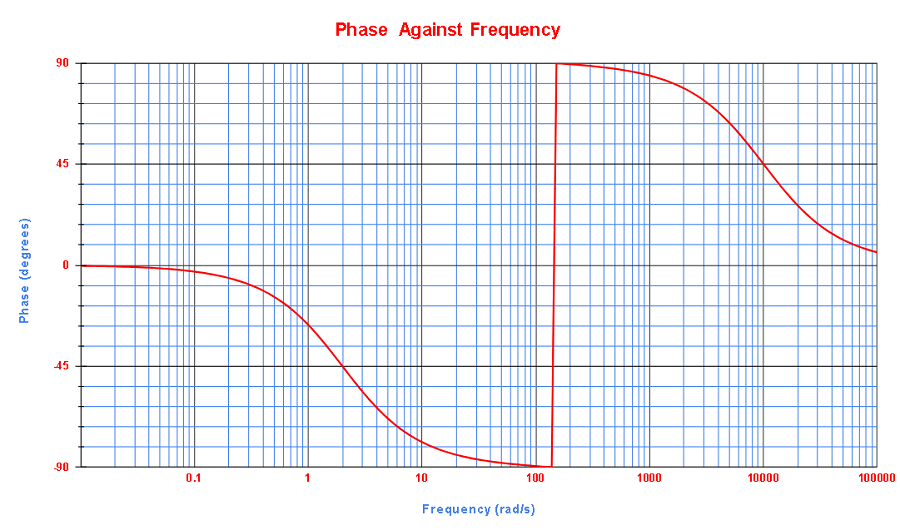Example 3
Find the transfer function in the frequency domain of the circuit below and graph it magnitude and argument (or phase).Solution to Example 3
To make manipulation of expressions easier, let $s = j \omega$ and express the impedances of the capacitors $C_1$ and $C_2$ in terms of $s$ as follows
$Z_{C_1} = \dfrac{1}{j \; \omega \; C_1} = \dfrac{1}{s C_1}$
and
$Z_{C_2} = \dfrac{1}{j \; \omega \; C_2} = \dfrac{1}{s C_2}$

We now use Kirchhoff's current and voltage and Ohm's law to write the equations
$I = I_1 + I_2 \qquad (I)$   Kirchhoff's current law at upper node
$V_{in} = R_1 I + Z_{c_1} I_1 \qquad (II)$   Kirchhoff's voltage law on closed loop on the left
$Z_{c_1} I_1 = (Z_{c_2} + R_2) I_2 \qquad (III)$   Kirchhoff's voltage law on closed loop on the right
$V_{out} = R_2 I_2 \qquad (IV)$   Ohm's law for voltage across $R_2$

Use euqations (II) and (IV) to write the transfer function $H(\omega )$ as follows
$H(s) = \dfrac{V_{out}}{V_{in}} = \dfrac{R_2 I_2}{R_1 I + Z_{c_1} I_1}$
Use equation (I) to substitute $I$ by $I_1 + I_2$ in $H(\omega)$ above
$H(s) = \dfrac{R_2 I_2}{R_1 ( I_1 + I_2) + Z_{c_1} I_1}$
Divide the numerator and denominator of the above by $I_2$, simplify and rewrite as
$H(s) = \dfrac{R_2}{R_1 \left( \dfrac{I_1}{I_2} + 1 \right) + Z_{c_1} \dfrac{I_1}{I_2}} \qquad (V)$
Use equation (III) to obtain
$\dfrac{I_1}{I_2} = \dfrac{Z_{c_2} + R_2}{Z_{c_1}}$

Substitute $\dfrac{I_1}{I_2}$ by $\dfrac{Z_{c_2} + R_2}{Z_{c_1}}$ in $(IV)$ and rearrange to obtain $H(\omega)$

$H(s) = \dfrac{R_2 Z_{c_1} }{(R_1 + Z_{c_1})(R_2 + Z_{c_2} ) + R_1 Z_{c_1}}$

We now substitute the capacitances given by their given numerical values to obtain
$Z_{c_1} = \dfrac{2 \cdot 10^4}{s}$ and $Z_{c_2} = \dfrac{10^4}{s}$
We now substitute to obtain
$H(s) = \dfrac{250 \times \dfrac{2 \cdot 10^4}{s} }{\left(100 + \dfrac{2 \cdot 10^4}{s}\right) \left(250 + \dfrac{10^4}{s} \right) + 100 \times \dfrac{2 \cdot 10^4}{s}}$
Simplify
$H(s) = \dfrac{ 200 s}{s^2 + 320 s + 8000}$
Substitute $s$ by $j\; \omega$
$H(\omega) = \dfrac{ j \; 200 \; \omega}{-\omega^2 + 8000 + j \; 320 \; \omega }$

$| H(\omega)| = \dfrac{200 \; \omega}{\sqrt {(8000 - \omega^2)^2 + (320 \; \omega)^2} }$

$\phi(\omega) = \dfrac{\pi}{2} - \arctan \left(\dfrac{320 \; \omega}{-\omega^2 + 8000} \right)$
The graphs of $\; 20 \log_{10} | H(\omega) |$ and the phase $\phi(\omega)$ are shown below.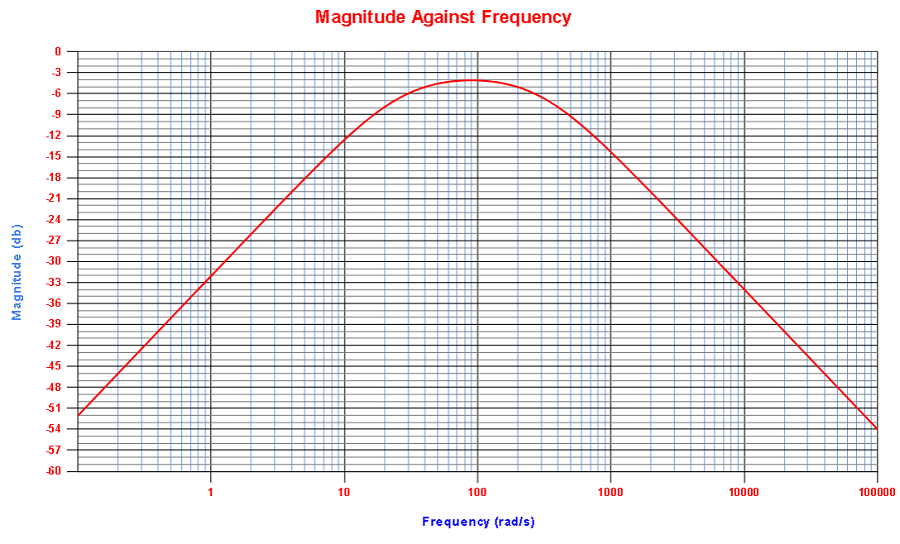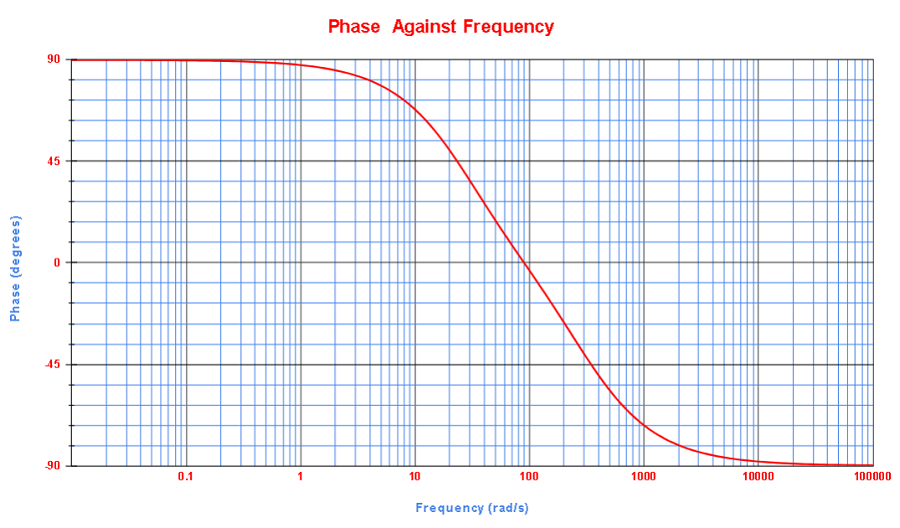## Problems with Solutions

Find the transfer function in the frequency domain for each circuit below in parts A and B.
Part A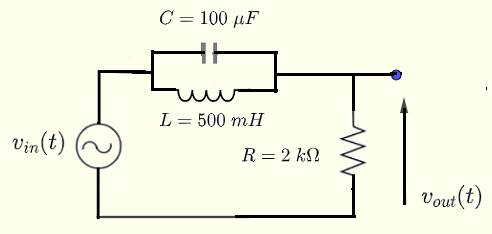Part B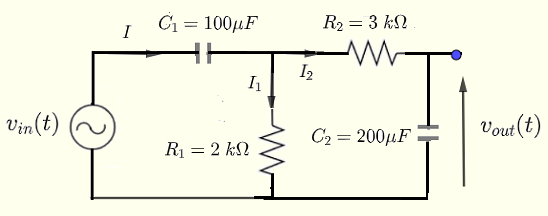Part C
Apply the formula of two cascaded circuits to find the transfer function, in the frequency domain, of the circuit shown below.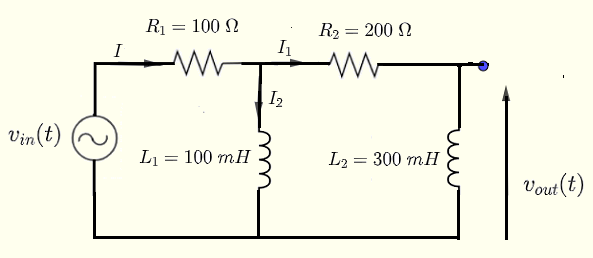## Solutions to the Above Problems

Part A
Let $s = j \; \omega$ , $Z_C = \dfrac{1}{C \; s}$ the impedance of the capacitor $C$ and $Z_L = L \; s$ the impedance of the inductor $L$.
The impedance $Z$ equivalent to $Z_C$ parallel to $Z_L$ is given by
$\dfrac{1}{Z} = \dfrac{1}{Z_C} + \dfrac{1}{Z_L}$
which may be written as
$Z = \dfrac{Z_C \; Z_L}{ Z_C + Z_L }$
The voltage $V_{out}$ is given by
$V_{out} = \dfrac { V_{in}}{ Z + R } R$
The transfer function is given by
$H(s) = \dfrac{V_{out}}{V_{in}} = \dfrac{R}{Z + R}$
Calculate $Z$ in terms of $C$ and $L$.
$Z = \dfrac{ \dfrac{1}{C \; s} \; L \; s }{ \dfrac{1}{C \; s} + L \; s } = \dfrac{L \; s}{ 1 + L \; C \; s^2}$

Substitute $Z$ by its expression above in $H(\omega)$ to obtain
$H(s) = \dfrac{R (1 + L \; C \; s^2)}{L \; s + R \; (1 + L \; C \; s^2)} \\\\ \quad = \dfrac{R\;L\;C \; s^2 + R}{R\;L\;C s^2 + L\;S + R}$

Substitute $s = j \omega$ to obtain
$H(\omega) = \dfrac{R\;L\;C \; \omega^2 + R}{-R\;L\;C \; \omega^2 + R + j \; \omega L }$

Part B
Let $s = j \omega$ and express the impedances of the capacitors $C_1$ and $C_2$ as follows
$Z_{C_1} = \dfrac{1}{j \; \omega \; C_1} = \dfrac{1}{s C_1}$
and
$Z_{C_2} = \dfrac{1}{j \; \omega \; C_2} = \dfrac{1}{s C_2}$
Use Kirchhoff's current and voltage and Ohm's law to write 4 equations in a very similar way as was done in example 3 above and solve to obtain the transfer function.
$H(s) = \dfrac{R_1 \; C_1 \; s }{(R_1 \; C_1 \; s + 1)(R_2 \; C_2 \; s + 1) + R_1 \; C_2 \; s } \qquad (I)$

$R_1 C_1 = 2 \cdot 10^3 \times 100 \cdot 10^{-6} = \dfrac{1}{5}$

$R_2 C_2 = 3 \cdot 10^3 \times 200 \cdot 10^{-6} = \dfrac{3}{5}$

$R_1 \; C_2 = 2 \cdot 10^3 \times 200 \cdot 10^{-6} = \dfrac{2}{5}$
Substitute $R_1 \; C_1$ and $R_2 \; C_2$ by their numerical values, expand the denominator and substitute $s$ by $j \; \omega$ in (I) above to obtain the transfer function in the $s$ and the frequency domains.
$H(s) = \dfrac{ 5 s }{ 3 s^2 + 30 s + 25 }$

$H(\omega) = \dfrac{ j \; 5 \;\omega }{ - 3 \; \omega^2 + 25 + j \; 30 \; \omega }$

Part C

$H(s) = \dfrac{Z_4 Z_2 }{(Z_1 + Z_2)(Z_4 + Z_3 ) + Z_1 Z_2}$

We now calculate the impedances $Z_1, Z_2, Z_3$ and $Z_4$ using the given numerical values in the circuit in part b).
$Z_1 = 100$ , $Z_2 = 0.1 s$ , $Z_3 = 200$ , $Z_4 = 0.3 s$
We now substitute to obtain
$H(s) = \dfrac{0.03 \; s^2}{(100 + 0.1 \; s)(0.3 s + 200 ) + 10 \; s}$
Simplify
$H(s) = \dfrac{3 \; s^2}{3 \; s^2 + 6000 \; s + 2000000}$
Substitute $s$ by $j\; \omega$
$H(\omega) = \dfrac{ - \; 3 \; \omega^2}{- 3 \omega^2 + 2000000+ j \; 6000 \; \omega }$